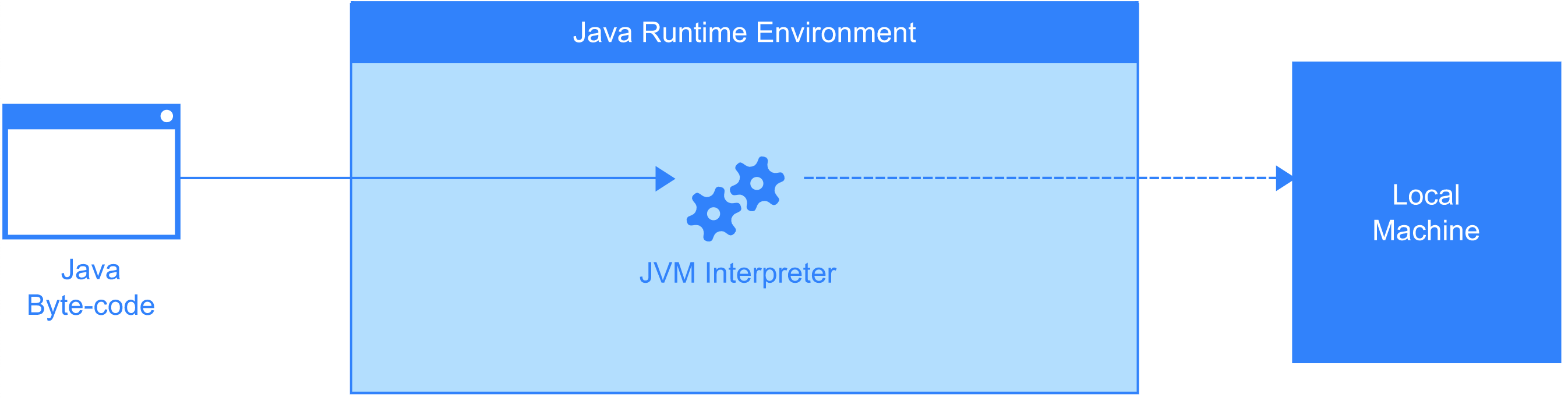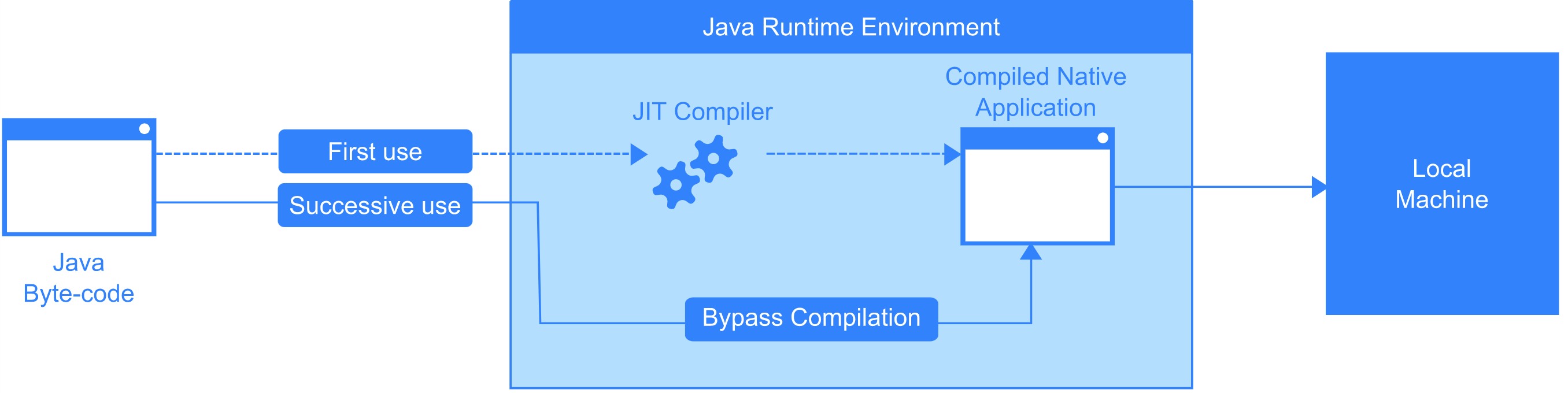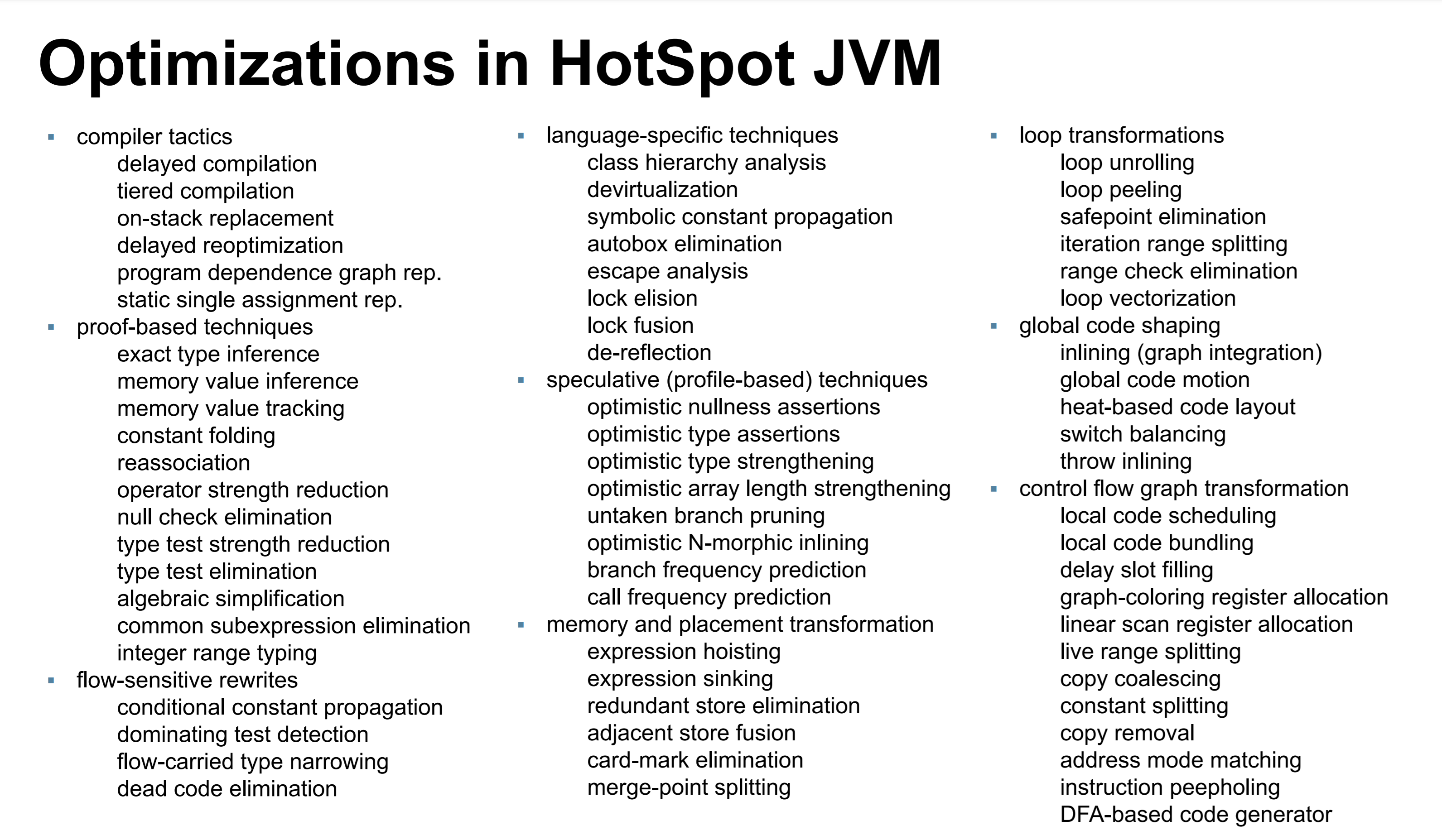# #### 一个 println 竟然比 volatile 还好使？jvm
java
1周前

``````static boolean stopRequested = false;

public static void main(String[] args) throws InterruptedException {

Thread backgroundThread = new Thread(() -> {
int i = 0;
while (!stopRequested) {
i++;
}
}) ;
backgroundThread.start();
TimeUnit.MICROSECONDS.sleep(10);
stopRequested = true ;
}
``````

``````static boolean stopRequested = false;

public static void main(String[] args) throws InterruptedException {

Thread backgroundThread = new Thread(() -> {
int i = 0;
while (!stopRequested) {

// 加上一行打印，循环就能退出了！
System.out.println(i++);
}
}) ;
backgroundThread.start();
TimeUnit.MICROSECONDS.sleep(10);
stopRequested = true ;
}
``````## JIT（Just-in-Time） 的优化### 表达式提升（expression hoisting）

``````public void hoisting(int x) {
for (int i = 0; i < 1000; i = i + 1) {
// 循环不变的计算
int y = 654;
int result = x * y;

// ...... 基于这个 result 变量的各种操作
}
}
``````

``````public void hoisting(int x) {
int y = 654;
int result = x * y;

for (int i = 0; i < 1000; i = i + 1) {
// ...... 基于这个 result 变量的各种操作
}
}
``````

``````static boolean stopRequested = false;// 静态变量

public static void main(String[] args) throws InterruptedException {

Thread backgroundThread = new Thread(() -> {
int i = 0;
while (!stopRequested) {
// leaf method
i++;
}
}) ;
backgroundThread.start();
TimeUnit.MICROSECONDS.sleep(10);
stopRequested = true ;
}
``````

``````int i = 0;

boolean hoistedStopRequested = stopRequested;// 将stopRequested 提升为局部变量
while (!hoistedStopRequested) {
i++;
}
``````

### 表达式下沉（expression sinking）

``````public void sinking(int i) {
int result = 543 * i;

if (i % 2 == 0) {
// 使用 result 值的一些逻辑代码
} else {
// 一些不使用 result 的值的逻辑代码
}
}
``````

``````public void sinking(int i) {
if (i % 2 == 0) {
int result = 543 * i;
// 使用 result 值的一些逻辑代码
} else {
// 一些不使用 result 的值的逻辑代码
}
}
``````

## JIT 还有那些常见优化？

### 循环展开（Loop unwinding/loop unrolling）

``````for (int i = 0; i < 100000; i++) {
delete(i);
}
``````

``````for (int i = 0; i < 20000; i+=5) {
delete(i);
delete(i + 1);
delete(i + 2);
delete(i + 3);
delete(i + 4);
}
``````

### 内联优化（Inling）

JVM 的方法调用是个栈的模型，每次方法调用都需要一个压栈（push）和出栈（pop）的操作，编译器也会对调用模型进行优化，将一些方法的调用进行内联。

``````public  void inline(){
int a = 5;
int b = 10;
int c = calculate(a, b);

// 使用 c 处理……
}

public int calculate(int a, int b){
return a + b;
}
``````

``````public  void inline(){
int a = 5;
int b = 10;
int c = a + b;

// 使用 c 处理……
}
``````

### 提前置空

``````class A {
// 对象被回收前，会触发 finalize
@Override protected void finalize() {
System.out.println(this + " was finalized!");
}

public static void main(String[] args) throws InterruptedException {
A a = new A();
System.out.println("Created " + a);
for (int i = 0; i < 1_000_000_000; i++) {
if (i % 1_000_00 == 0)
System.gc();
}
System.out.println("done.");
}
}

//打印结果
Created A@1be6f5c3
A@1be6f5c3 was finalized!//finalize方法输出
done.
``````

## HotSpot VM JIT 的各种优化项## 参考

1438 阅读

### 评论列表burnbrid2天前